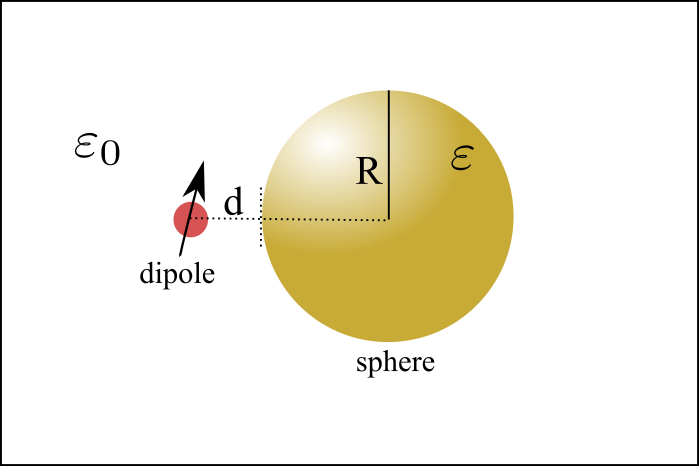# Dipole’s Emission Near Sphere

## IntroductionAs is shown in above figure, In this project I will simulate the dipole’s emission near a sphere. The sphere could be dielectric or metalic. The theoretic description could be found in this paper ref1.

We will analyze two different types of dipole orientations.

• Radial dipole orientation which means dipole is orthogonal to the radial direction. $\mathrm{d}_{ort}$
• Tangential dipole orientation which means dipole is parallel to the radial direction. $\mathrm{d}_{para}$

We will try to numerically and theoretically calculate the emission enhancement.

## Theoretical Description

Theoretically, the total decay rate $\Gamma{t}$ and radiative decay rate $\Gamma{r}$ could be expressed as

• Tangential Dipole

In above expressions, $j{n},h^{(1)}{n}$ are ordinary spherical Bessel and Hankel functions.

$a{n}$ and $b{n}$ are the Mie scattering coefficients of the sphere. $r=R+d$, $k=\sqrt{\varepsilon}\omega/c$. $\varepsilon$ is the dielectric constant of the embedding medium, $\omega$ the optical frequency, $c$ the speed of the light in vacuum, and $l$ is the angular mode number. The derivatives of $\psi{n},\xi{n}$ are relatively to $kr$. The Mie scattering coefficients’ definition could be found in the book.ref2

### MATLAB Implementation

I wrote an MATLAB program to realize the simulation of the emission enhancement. The following is the function and these function should be renamed and stored with a proper name according to the function name.

#### Main Program (Convergence Test)

And the main program is as follows

### Python Implementation

I also write a corresponding python program to calculate the dipole’s emission near sphere.

## COMSOL Simulation

Dipole’s emission properties near nano particles could be simulated via COMSOL. COMSOL supports different types of sources, for example, the point, edge, surface and volume current source. The emission power could be described by

to be continued…….

## Lumerical FDTD Simulations

ref1. Phys. Rev. B 76, 115123 (2007)
ref2. Absorption and scattering by small particles